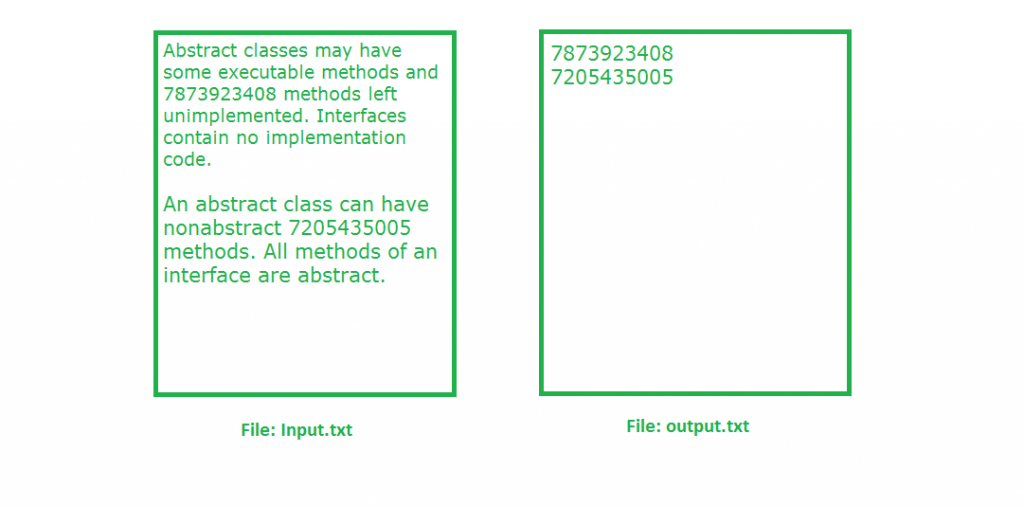Open In App

# Java program to read all mobile numbers present in given file

Given the input text file, read all mobile numbers present and write to some output file.

Suppose we have a file names as input.txt and in that input file we have some Mobile Number which are mixed with some other data. Our task is to read the input file line by line and if we find any Number in that line we have to write that Mobile Number to an another file, which is output.txt file.

Example :Mobile Number validation criteria :

• The first digit should contain number between 7 to 9.
• The rest 9 digit can contain any number between 0 to 9.
• The mobile number can have 11 digits also by including 0
at the starting.
• The mobile number can be of 12 digits also by including 91
at the starting

The number which satisfies the above criteria, is a valid mobile Number.

Approach : We use Regular expression, first we have to form a regular expression for Mobile Number. Whenever any Number in the input.txt file matches with that regular expression which we form for Mobile Number, that matched number will be written to output.txt file.

Below is Java implementation is above approach :

 `// Java program to read all mobile numbers``// present in given file``import` `java.util.regex.*;``import` `java.io.*;`` ` `class` `MobileNumberExtraction ``{``    ``public` `static` `void` `main(String[] args) ``throws` `IOException``    ``{``        ``// Write Mobile Numbers to output.txt file``        ``PrintWriter pw = ``new` `PrintWriter(``"output.txt"``);`` ` `        ``// Regular expression for mobile number``        ``Pattern p = Pattern.compile(``"(0/91)?[7-9][0-9]{9}"``);`` ` `        ``// BufferedReader for reading from input.txt file``        ``BufferedReader br = ``new` `BufferedReader``                                ``(``new` `FileReader(``"input.txt"``));``        ``String line = br.readLine();``         ` `        ``while` `(line != ``null``) ``        ``{``            ``Matcher m = p.matcher(line);`` ` `            ``while` `(m.find()) ``            ``{``                ``// Write the mobile number to output.txt file``                ``pw.println(m.group());``            ``}`` ` `            ``line = br.readLine();``        ``}``        ``pw.flush();``    ``}``}`

Output :

```7873923408
7205435005
```

The above output is for this input.txt file :

```Abstract classes may have some executable methods
and 7873923408 methods left unimplemented. Interfaces
contain no implementation code. An abstract
class can have non abstract 7205435005 methods.
All methods 1234 of an interface are abstract.```